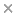BaseSpace
Correlation Engine 2.0Search sequence regions

###### filter terms:
Sizes of these terms reflect their relevance to your search.

Single nucleotide polymorphisms (SNPs) are the most common form of genetic variation in human DNA. The sequence of SNPs in each of the two copies of a given chromosome in a diploid organism is referred to as a haplotype. Haplotype information has many applications such as gene disease diagnoses, drug design, etc. The haplotype assembly problem is defined as follows: Given a set of fragments sequenced from the two copies of a chromosome of a single individual, and their locations in the chromosome, which can be pre-determined by aligning the fragments to a reference DNA sequence, the goal here is to reconstruct two haplotypes (h1, h2) from the input fragments. Existing algorithms do not work well when the error rate of fragments is high. Here we design an algorithm that can give accurate solutions, even if the error rate of fragments is high. We first give a dynamic programming algorithm that can give exact solutions to the haplotype assembly problem. The time complexity of the algorithm is O(n × 2t × t), where n is the number of SNPs, and t is the maximum coverage of a SNP site. The algorithm is slow when t is large. To solve the problem when t is large, we further propose a heuristic algorithm on the basis of the dynamic programming algorithm. Experiments show that our heuristic algorithm can give very accurate solutions. We have tested our algorithm on a set of benchmark datasets. Experiments show that our algorithm can give very accurate solutions. It outperforms most of the existing programs when the error rate of the input fragments is high.

### Citation

Fei Deng, Wenjuan Cui, Lusheng Wang. A highly accurate heuristic algorithm for the haplotype assembly problem. BMC genomics. 2013;14 Suppl 2:S2

PMID: 23445458

View Full Text